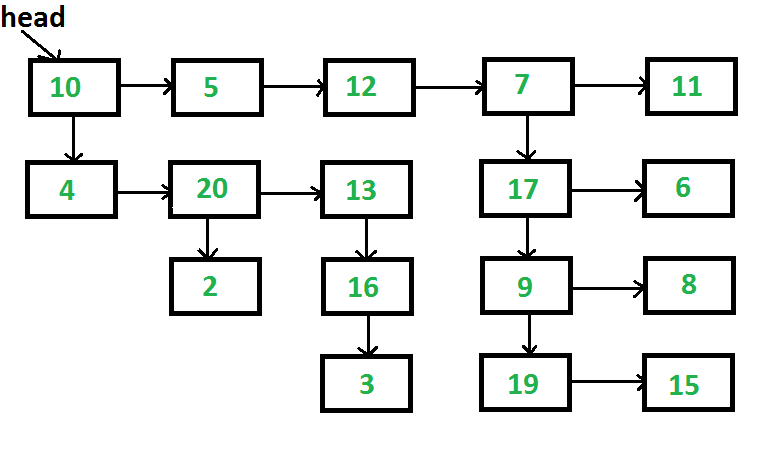GeeksforGeeks App
Open AppBrowser
Continue

# Python Program For Flattening A Multilevel Linked List

Given a linked list where in addition to the next pointer, each node has a child pointer, which may or may not point to a separate list. These child lists may have one or more children of their own, and so on, to produce a multilevel data structure, as shown in the below figure. You are given the head of the first level of the list. Flatten the list so that all the nodes appear in a single-level linked list. You need to flatten the list in a way that all nodes at the first level should come first, then nodes of the second level, and so on.
Each node is a C struct with the following definition.

## Python3

 `# A linked list node has data,``# next pointer and child pointer``class` `Node:``    ``def` `__init__(``self``, data):``        ``self``.data ``=` `data``        ``self``.``next` `=` `None``        ``self``.child ``=` `None``        ` `        ``# This code contributed by umadevi9616`The above list should be converted to 10->5->12->7->11->4->20->13->17->6->2->16->9->8->3->19->15

The problem clearly says that we need to flatten level by level. The idea of a solution is, we start from the first level, process all nodes one by one, if a node has a child, then we append the child at the end of the list, otherwise, we don’t do anything. After the first level is processed, all next-level nodes will be appended after the first level. The same process is followed for the appended nodes.

```1) Take the "cur" pointer, which will point to the head
of the first level of the list
2) Take the "tail" pointer, which will point to the end of
the first level of the list
3) Repeat the below procedure while "curr" is not NULL.
I) If the current node has a child then
a) Append this new child list to the "tail"
tail->next = cur->child
b) Find the last node of the new child list and update
the "tail"
tmp = cur->child;
while (tmp->next != NULL)
tmp = tmp->next;
tail = tmp;
II) Move to the next node. i.e. cur = cur->next```

Following is the implementation of the above algorithm.

## Python3

 `# Python3 Program to flatten list with``# next and child pointers` `# A linked list node has data,``# next pointer and child pointer``class` `Node:``    ``def` `__init__(``self``, data):``        ``self``.data ``=` `data``        ``self``.``next` `=` `None``        ``self``.child ``=` `None` `# Return Node``def` `newNode(data):``    ``return` `Node(data)` `# The main function that flattens``# a multilevel linked list``def` `flattenlist(head):``    ` `    ``# Base case``    ``if` `not` `head:``        ``return``    ` `    ``# Find tail node of first level``    ``# linked list``    ``temp ``=` `head``    ``while``(temp.``next` `!``=` `None``):``        ``temp ``=` `temp.``next``    ``currNode ``=` `head``    ` `    ``# One by one traverse through all``    ``# nodes of first level linked list``    ``# till we reach the tail node``    ``while``(currNode !``=` `temp):``        ` `        ``# If current node has a child``        ``if``(currNode.child):``            ` `            ``# then append the child``            ``# at the end of current list``            ``temp.``next` `=` `currNode.child``            ` `            ``# and update the tail to new``            ``# last node``            ``tmp ``=` `currNode.child``            ``while``(tmp.``next``):``                ``tmp ``=` `tmp.``next``            ``temp ``=` `tmp``            ` `        ``# Change current node``        ``currNode ``=` `currNode.``next``    ` `# A utility function to print``# all nodes of a linked list``def` `printList(head):``    ``if` `not` `head:``        ``return``    ``while``(head):``        ``print``(``"{}"``.``format``(head.data),``              ``end ``=` `" "``)``        ``head ``=` `head.``next` `# Driver code``if` `__name__``=``=``'__main__'``:``    ` `    ``# Child list of 13``    ``child13 ``=` `newNode(``16``)``    ``child13.child ``=` `newNode(``3``)``    ` `    ``# Child List of 10``    ``head1 ``=` `newNode(``4``)``    ``head1.``next` `=` `newNode(``20``)` `    ``# Child of 20``    ``head1.``next``.child ``=` `newNode(``2``)``    ``head1.``next``.``next` `=` `newNode(``13``)``    ``head1.``next``.``next``.child ``=` `child13``    ` `    ``# Child of 9``    ``child9 ``=` `newNode(``19``)``    ``child9.``next` `=` `newNode(``15``)` `    ``# Child List of 17``    ``child17 ``=` `newNode(``9``)``    ``child17.``next` `=` `newNode(``8``)``    ``child17.child ``=` `child9` `    ``# Child List of 7``    ``head2 ``=` `newNode(``17``)``    ``head2.``next` `=` `newNode(``6``)``    ``head2.child ``=` `child17``    ` `    ``# Main List``    ``head ``=` `newNode(``10``)``    ``head.child ``=` `head1``    ``head.``next` `=` `newNode(``5``)``    ``head.``next``.``next` `=` `newNode(``12``)``    ``head.``next``.``next``.``next` `=` `newNode(``7``)``    ``head.``next``.``next``.``next``.child ``=` `head2``    ``head.``next``.``next``.``next``.``next` `=` `newNode(``11``)` `    ``flattenlist(head)` `    ``print``(``"Flattened list is: "``, end ``=` `"")``    ``printList(head)``# This code is contributed by 0_hero`

Output:

`10 5 12 7 11 4 20 13 17 6 2 16 9 8 3 19 15`

Time Complexity: Since every node is visited at most twice, the time complexity is O(n) where n is the number of nodes in given linked list.

Space Complexity : O(1)

Please refer complete article on Flatten a multilevel linked list for more details!

My Personal Notes arrow_drop_up